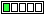All about flooble | fun stuff | Get a free chatterbox | Free JavaScript | Avatarsperplexus dot infoLegally Blonde (Posted on 2016-12-19)You meet three blonde women, A, B, and C. Each of them is either a knight or a liar. Exactly one of them is Elle Woods.

A:I am Elle Woods.
B:I am Elle Woods.
C:At least two of us are liars.

What are A, B, and C, and who is Elle Woods?

 No Solution Yet Submitted by Math Man Rating: 3.0000 (1 votes)Comments: ( Back to comment list | You must be logged in to post comments.)Can hair be lying?| Comment 6 of 8 |Evidentally, A or B (or both) must be a liar.
Suppose C is also a liar. Then C's statement would be true, which is not possible. Since therefore C must be a knight, it follows from C's statement that A and B are both liars.
So only C can be Elle Woods.
(But maybe all of them are implicitly lying about the colour of their hair.)

SOLUTION
------------

?- blondewomen(A,B,C).

A = a(0,0),
B = b(0,0),
C = c(1,1)

PROGRAM
-----------
:- op(1050, xfy, =>).           % operator definition '=>'

X => Y :- \+ X, !.                % de Morgan: (P -> Q) <=> (~P v Q)
X => Y :- Y.

statement(1).
statement(0).
ellewoods(1).
ellewoods(0).

blondewomen(a(S1,E1),b(S2,E2),c(S3,E3)) :-

statement(S1),statement(S2),statement(S3),
ellewoods(E1),ellewoods(E2),ellewoods(E3),

TruthSum is (S1+S2+S3),

((S1=1) => (E1=1)),
((S2=1) => (E2=1)),
((S3=1) => (TruthSum =<1)),

((S1=0) => \+ (E1=1)),
((S2=0) => \+ (E2=1)),
((S3=0) => \+ (TruthSum =<1)),

EW_identitySum is (E1+E2+E3),
EW_identitySum is 1.

 Posted by ollie on 2016-12-19 14:51:11Please log in:

 Search: Search body:
Forums (0)